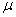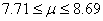Self Test 6 for Probability and Statistics

## Estimation Theory

This test was constructed by Zauresh Atakhanova based on the Schaum's Outline Theory and Problems of Probability and Statistics by Murray R. Spiegel with contributions from others as noted below. If you need more review refer also to this outline.

## Unbiased and Efficient Estimates

1. True False Give an example of estimators (or estimates) which are (a) unbiased and efficient, (b) unbiased and inefficient, (c) biased and inefficient.

a)     sample mean

b)     sample median

c)     sample standard deviation

Confidence Interval Estimates for Means (Large Samples)

2.True False. Measurements of the diameters of a random sample of 196 ball bearings made by a certain machine during one week showed a mean of 0.724 inches and a standard deviation of 0.042. Find (a) 95% and (b) 99% confidence interval for the mean diameter of all the ball bearings.

a) 0.724+/-0.0077

b) 0.724+/-0.0058

Confidence Interval Estimates for Means (Small Samples)

3.True False. The 95% confidence coefficients (1.96 'two-tailed') for the normal distribution are given by +/-1.96. What are the corresponding coefficients for the t distribution if (a) v=9, (b) v=30?

(a) +/-2.26

(b) +/-2.04

## Confidence Interval Estimates for Proportions

4.True False. A sample poll of 1000 voters chosen at random from all voters in a given district indicated that 45% of them were in favor of a particular candidate. Find (a) 95%, (b) 99% and (c) 99.73% confidence limits for the proportion of all the voters in favor of this candidate.

(a)    0.45+/-0.0106

(b)   0.45+/-0.0157

(c)    0.45+/-0.0472

## Confidence Intervals for Difference and Sums

5.True False. A sample of 150 brand A light bulbs showed a mean lifetime of 1500 hours and a standard deviation of 1300 hours. A sample of 200 brand B light bulbs showed a mean lifetime of 1200 hours and a standard deviation of 80 hours. Find (a) 95% and (b) 99% confidence limits for the difference of the mean lifetimes of the populations of brands A and B.

a)     200+/-20.8

b)     200+/-28.6

# Confidence Intervals for Variances

6.True False. The standard deviation of the lifetimes of a sample of 200 electric light bulbs was computed to be 150 hours. Find (a) 95% and (b) 99% confidence intervals for the standard deviation of all such light bulbs.

a)     150+/-5.3

b)     150+/-8.7

7.True False.Work problem 5 using small sampling theory.

(a)    (136.86, 167.03)

(b)   (133.42, 173.88)

# Confidence Intervals for Variance Ratios

8.True False. Two samples of sizes 15 and 10 are drawn at random from two normal populations. If their variances are found to be 24 and 16 respectively, with 90% confidence interval for the ratio of the variances is (0.4804, 3.7451)

9. True False A copper smelter in northern Chile receives copper concentrate from 50 different small operations in the area with varying grades. On average the smelter receives concentrates grading at 85% Cu with a standard deviation of 5% Cu. The company that owns the smelter can be 95% confident that its operation receives a concentrate grade between 83.6% and 86.5%. (Contributed by J. Mazumdar)

More Confidence Interval Estimates for Means (Large Samples)

10. True False After extensive test drilling of on area of possible gold ore, a random sample of 200 Kg. Drill core were measured by a machine to find their gold content. The sampling showed a mean of 8.24g of gold per sample with a standard deviation of 0.42g. Find (a) 95% and (b) 99% confidence interval for the mean gold content of all the samples.

a)     8.24 + 0.058g

b)     8.24 + 0.77g

11. True False A sample of 200 employees has found that the average number of days off sick in a year was 8.2, with a standard deviation of 3.5. Then the average number of days off sick to be for the employed population is the 95% confidence interval forwhich is. (Contributed by Mansoor Al-Harthy)

12. True False Currently, environmental regulations allow your factory to have maximum indoor emissions of particular matter (PM-2.5) of an annual mean concentration of 40 mg/m3 (arithmetic mean). Local inspectors collected measurements from your plant 18 times last year; these measurements resulted in a mean concentration of PM-2.5 of 45 mg/m3 with a sample standard deviation of 8. Assuming that the concentration of PM-2.5 from your plant follows a normal distribution, the inspectors correctly concluded with 99% confidence that your plant last year was in violation of the environmental regulations and you should be fined accordingly. (Contributed by Alex Lombardia)